# Electrochemistry Class 12 Notes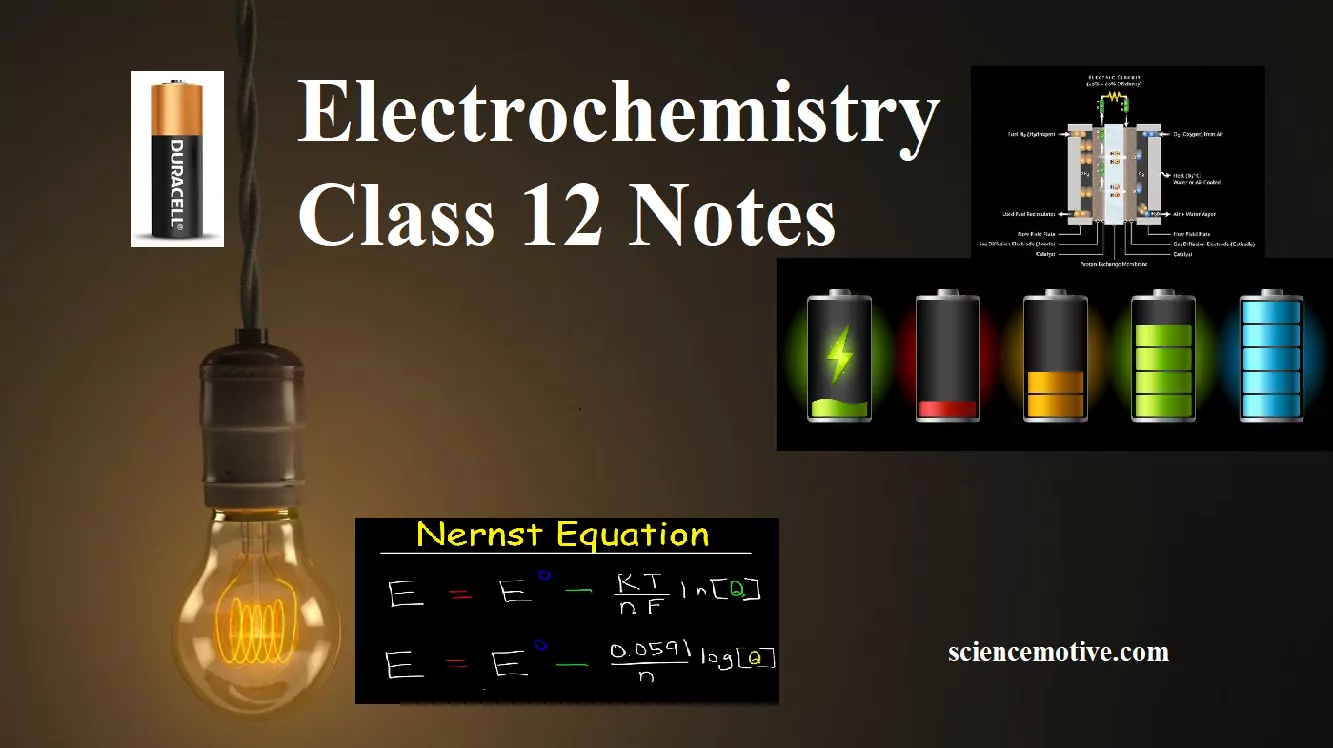Electrochemistry Class 12 Notes

Syllabus:-

• Electrolytic cells & Galvanic cells
• The function of Salt Bridge
• Redox reaction, EMF of the cell, standard electrode potential
• Standard hydrogen electrode (SHE)
• Nernst equation and its application to Chemical cell
• Equilibrium Constant from Nernst Equation
• Electrochemical Cell and Gibbs Energy

Electrochemistry: It is a branch of chemistry that deals with the relationship between chemical energy and electrical energy and their interconversions.

Redox Reactions: Oxidation is the process that involves the loss of electrons & reduction is a process in which it involves the gain of electrons. The reactions which involve both that reaction simultaneously are called a redox reaction.

Electrochemical Cells: These are devices that convert the chemical energy of some redox reactions to electrical energy. They are also called Galvanic cells or Voltaic cells. An example for a Galvanic cell is Daniel cell.

It is constructed by dipping a Zn rod in ZnSO4 solution and a Cu rod in CuSO4 solution. The two solutions are connected externally by a metallic wire through a voltmeter and a switch and internally by a salt bridge.

A salt bridge is a U-tube containing an inert electrolyte like NaNO3 or KNO3 in a gelly like substance. The functions of a salt bridge are:

1. To complete the electrical circuit
2. To maintain the electrical neutrality in the two half cells.
• Cu2+ + 2 e → Cu(s) (reduction half reaction)
• Zn(s) → Zn2+ + 2 e (oxidation half reaction)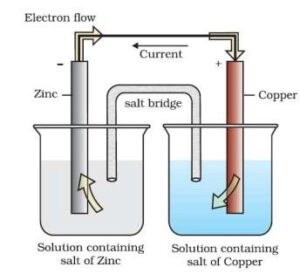These reactions occur in two different portions of the Daniel cell. The reduction half-reaction occurs on the copper electrode while the oxidation half-reaction occurs on the zinc electrode. These two portions of the cell are also called half-cells or redox couples. The copper electrode may be called the reduction half-cell and the zinc electrode, the oxidation half-cell.

Electrochemistry Class 12 Notes

Electrode Potential: This tendency of a metal to lose or gain an electron when it is in contact with its own solution is called electrode potential.

Standard electrode potential (E0): The electrode potential measured at standard conditions.ie at 298K,1 atm pressure, and at 1 molar concentration.

Standard hydrogen electrode (SHE): The reference electrode used to measure single electrode potential. Its potential is assumed to be zero. It consists of a platinum wire dipped in HCl of 1 molar concentration. Hydrogen gas at 1 atm. is passed through the solution. The electrode can be represented as Pt, H2 /H+(1M).

Nernst Equation For A Cell Reaction:-
Let us consider a general equation
aA   +   bB →  cC   +   dD
E = E0cell  –   2.303 RT × log [C]c [D]d
nF                 [A]a [B]b
Where E0 is the standard electrode potential,
R is the gas constant (8.314 JK–1 mol–1)
F is Faraday constant (96500 C mol–1)
T is the temperature in Kelvin.

Nernst equation can be written as:
Putting the above values Nernst equations can be written as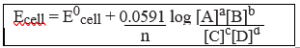Equilibrium Constant from Nernst Equation: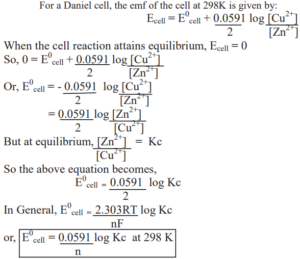Electrochemistry Class 12 Notes

Electrochemical Cell and Gibbs Energy of the Reaction:
Electrical work done in one second is equal to electrical potential multiplied by total charge passed. The reversible work done by a galvanic cell is equal to a decrease in its Gibbs energy and therefore if the emf of the cell is E and nF is the amount of charge passed and ∆G is the Gibbs energy of the reaction, then
∆G = –  nFEcell
If the concentration of all the reacting species is unity, then Ecell = E0cell and we have
∆G0 = – nFE0 cell
Thus, from the measurement of E0cell, we can calculate the standard Gibbs energy of the reaction.

Conductivity Molar conductivity and Electrolysis

Resistance (R): The electrical resistance is a hindrance to the flow of electrons. Its unit is the ohm (Ω). The resistance of a conductor is directly proportional to the length of the conductor (Ɩ) and inversely proportional to the area of cross-section (A) of the conductor.
R α Ɩ /A
R = ρ Ɩ /A
where ρ (rho) is a constant called resistivity or specific resistance. Its unit is ohm-meter (Ω m) or ohm-centimeter (Ω cm).

Resistivity (ρ): It defined as the resistance offered by a conductor having unit length and unit area of cross-section.

Conductance (G): It is the inverse of resistance.
i.e. G = 1/R. Its unit is ohm-1 or mho or Siemens (S)

Measurement of the conductivity of ionic solutions:
We know that conductivity G = ƙ × A/ Ɩ
So conductivity, ƙ = G × Ɩ/A
The quantity Ɩ/A is called cell constant (G*). It depends on the distance between the electrodes and their area of cross-section. Its unit is m-1.
i.e. Conductivity = Conductance × Cell Constant

Molar conductivity (λm):
It is the conductivity of 1 mole of an electrolytic solution kept between two electrodes with a unit area of cross-section and at a distance of unit length. It is related to the conductivity of the solution by the equation,
λm= ƙ/C (where C is the concentration of the solution)
Or,        λm = 1000 ƙ/M (where M is the molarity)
The unit of molar conductivity is Ω-1 cm2 mol-1 or S cm2 mol-1.

Variation of conductivity and Molar conductivity with concentration:
Both conductivity and molar conductivity change with the concentration of the electrolyte. For both strong and weak electrolytes, conductivity always decreases with dilution. This is because conductivity is the conductance of the unit volume of the electrolytic solution. As dilution increases, the number of ions per unit volume decreases, and hence the conductivity decreases.
For strong electrolytes, as dilution increases, the force of attraction between the ions decreases and hence the ionic mobility increases. So molar conductivity increases. When dilution reaches maximum or concentration approaches zero, the molar conductivity becomes maximum and it is called the limiting molar conductivity (λ0m).

The variation of λm for strong and weak electrolytes is shown in the following graphs: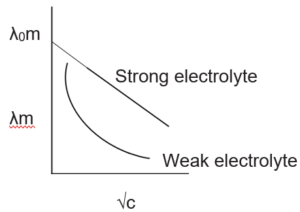For strong electrolytes, the value of λ0m can be determined by the extrapolation of the graph. But for weak electrolytes, it is not possible since the graph is not a straight line. So their λ0m values are calculated by applying Kohlrausch’s law of independent migration of ions.

Electrochemistry Class 12 Notes

Kohlrausch’s law: Molar conductance at infinite dilution of a strong electrolyte is equal to the sum of molar ionic conductance of the cation and anion at infinite dilution.
eg: λ0m NaOH = λ0m Na+ + λ0m OH-

Applications of Kohlrausch’s law:
(i) Determination of λ0m of weak electrolytes:
By knowing the λ0m values of strong electrolytes, we can calculate λ0m of weak electrolytes. For e.g. we can determine the λ0m of acetic acid (CH3COOH) by knowing the λ0m of CH3COONa, NaCl, and HCl as follows:
λ0m (CH3COONa) = λ0CH3COO + λ0Na+  …………. (1)
λ0m (HCl) = λ0H+ + λ0 Cl- …………….. (2)
λ0m (NaCl) = λ0Na+ + λ0Cl- ………….. (3)
(1) + (2) + (3) gives:
λ0m (CH3COONa) + λ0m (HCl) – λ0m (NaCl) = λ0CH3COO + λ0Na+ + λ0H+ + λ0Cl – λ0Na+ – λ0Cl–  = λ0CH3COOH
(ii) Determination of degree of dissociation of weak electrolytes:
By knowing the molar conductivity at a particular concentration (λcm) and limiting molar conductivity (λ0m), we can calculate the degree of dissociation (α) as,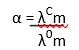By using α, we can calculate the dissociation constant of acid as: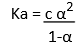Electrochemistry Class 12 Notes

Electrolytic Cells and Electrolysis: In an electrolytic cell, the electrical energy is converted to chemical energy. The dissociation of an electrolyte by the passage of electricity is called electrolysis. For e.g.
When CuSO4 solution is electrolyzed by Cu electrodes, Cu is deposited at the cathode and Cu2+ ions are liberated from the anode.

Quantitative Aspects of electrolysis – Faraday’s laws
The amount of substance(m) deposited at the electrode during electrolysis is directly proportional to the quantity of electricity(q) passed through the electrolyte.
m α Q
OR
m = ZQ
Where Z is a constant called electrochemical equivalent. Z = equivalent weight/96500
But the quantity of electricity is the product of current in ampere (I) and time in second (t).
i.e. Q = It
Therefore, m= Zit

When the same amount of electricity is passed through different electrolytic solutions, the amount of Substance deposited is proportional to the chemical equivalent weights.
For e.g. when the same quantity of electricity is passed through solutions of two substances A and B, then
Mass of A deposited = Equivalent mass of A
Mass of B deposited     Equivalent mass of B

Products of electrolysis:  The products of electrolysis depend on the nature of the electrolyte and the type of electrodes used. If the electrode is inert (e.g. Pt, gold, etc.), it does not participate in the electrode reaction. While if the electrode is reactive, it also participates in the electrode reaction.
For e.g. if molten NaCl is electrolyzed, Na is deposited at the cathode, and chlorine is liberated at the anode. NaCl → Na+ + Cl
At cathode: Na+ + e → Na
At anode: Cl → ½ Cl2 + e

Electrochemistry Class 12 Notes

Primary Secondary Batteries and Corrosion

Batteries: A battery is basically a galvanic cell in which the chemical energy of a redox reaction is converted to electrical energy. They are of mainly 2 types – primary batteries and secondary batteries.

Primary batteries: Here the reaction occurs only once and after use over a period of time, they become dead and cannot be reused. E.g. Dry cell, mercury button cell, etc. (Primary cells cannot be recharged and reused).

(i) Dry Cell   (ii) Mercury Cell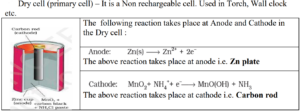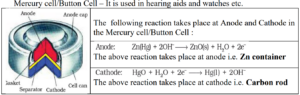Secondary cells: A secondary cell can be recharged and reused again and again. Here the cell reaction can be reversed by passing a current through it in the opposite direction. The most important secondary cell is the lead storage cell, which is used in automobiles and investors.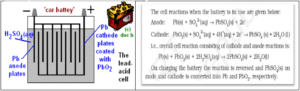Fuel Cells: These are galvanic cells that convert the energy of the combustion of fuels like hydrogen, methane, methanol, etc. directly into electrical energy.

One example of a fuel cell is the Hydrogen – Oxygen fuel cell, which is used in the Apollo space programme. Here hydrogen and oxygen are bubbled through porous carbon electrodes into a concentrated aqueous sodium hydroxide solution. To increase the rate of electrode reactions, catalysts like finely divided platinum or palladium metal are filled into the electrodes.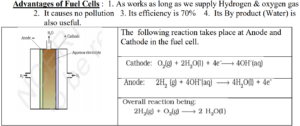Corrosion: It is a process of eating away of metals on their surfaces, it is an unwanted process as it results in a loss of mass of metals. In this process, metal surface reacts with atmospheric oxygen to form a layer of oxide. It is an electrochemical reaction.

The most familiar example of corrosion is the rusting of iron. It occurs in presence of water and air. It is a redox reaction. At a particular spot of the metal, oxidation takes place and that spot behaves as an anode. Here Fe is oxidized to Fe2+. 2 Fe (s)→2 Fe2+ + 4 e

Electrons released at the anodic spot move through the metal and go to another spot on the metal and reduce oxygen in presence of H+. This spot behaves as a cathode. The reaction taking place at this spot is:

The overall reaction is:  2Fe(s)+O2(g) + 4H+(aq) → 2Fe2 +(aq)+ 2 H2O (l )

The ferrous ions (Fe2+) are further oxidized to ferric ions (Fe3+) and finally to hydrated ferric oxide (Fe2O3. x H2O), which is called rust.

Methods to prevent corrosion:
(i) By coating the metal surface with paint, varnish, etc.
(ii) By coating the metal surface with another electropositive metal like zinc, magnesium, etc. The coating of metal with zinc is called galvanization and the resulting iron is called galvanized iron.
(iii) By coating with an anti-rust solution.
(iv) An electrochemical method is to provide a sacrificial electrode of another metal (like Mg, Zn, etc.) which corrodes itself but saves the object (sacrificial protection)

Electrochemistry Class 12 Notes